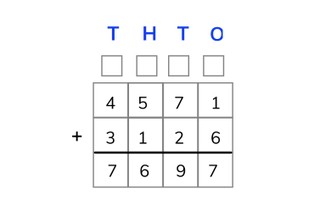Adding to 10,000 using the standard algorithm

# Adding to 10,000 using the standard algorithm

Students learn to add to 10,000 using the standard algorithm.8,000 schools use Gynzy92,000 teachers use Gynzy1,600,000 students use Gynzy

## General

The students can add to 10,000 using the standard algorithm with and without regrouping.

## Standards

CCSS.Math.Content.4.NBT.B.4

## Learning objective

Students will be able to add to 10,000 using the standard algorithm.

## Introduction

Have the students make the largest number they can with the numbers provided.

## Instruction

Check whether the students can add using the standard algorithm by asking the following questions:
- Why is it useful to add using the standard algorithm?
- What do the letters T H T O stand for?
- Where do you always begin when using the standard algorithm?
- Solve these problems by putting the numbers one under another and using the standard algorithm: 1428 + 273 = and 2569 + 3756

## Quiz

There are 10 exercises in which the students practice adding to 10,000 using the standard algorithm. For some problems the table is already (partially) filled in, and for others they must fill it in on their own. First the students practice several two-number problems with larger numbers, and then they practice two exercises with three numbers using the standard algorithm.

## Closing

Have the students drag the sums to the THTO-diagram and solve using the standard algorithm. Discuss again why it is useful to solve sums using the standard algorithm, and how you do this. Always work from right to left and use the boxes to carry over numbers for regrouping. This is also how you can solve problems with large numbers in quick steps without having to do the problem in your head.

## Teaching tips

For students that have difficulty using the standard algorithm, remind them of the meaning of T H T O and how you arrange the numbers in a THTO-diagram. Write out the intermediate sums. Note the ones values, tens numbers, hundreds numbers, and thousands numbers from the top to the bottom so it becomes obvious which numbers should be added together. Students that have already mastered using the standard algorithm can skip the instruction and may directly calculate sums using the standard algorithm without writing out intermediate sums.

### The online teaching platform for interactive whiteboards and displays in schools

• Save time building lessons

• Manage the classroom more efficiently

• Increase student engagement